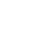# 数组写一个程序计算用户输入的数字的平均数

```每天早上七点三十推送干货数据可以存放在变量里，每一个变量有一个名字，有一个类型，还有它的生存空间。如果我们需要保存一些相同类型、相似含义、相同生存空间的数据，我们可以用数组来保存这些数据，而不是用很多个独立的变量。数组是长度固定的数据结构，用来存放指定的类型的数据。一个数组里可以有很多个数据，所有的数据的类型都是相同的。①创建数组数组是一种容器（放东西的地方）特点是：其中所有的元素具有相同的数据类型；一旦创建，不能改变大小数组中的每个数据叫做元素写一个程序计算用户输入的数字的平均数，并输出所有大于平均数的数。必须先记录每一个输入的数字，计算平均数之后，再检查记录下来的每一个数字，与平均数比较，决定是否输出。如何记录很多数？`int[]numbers=new int[]`

`public static void main(String[] args) {``    ``    Scanner in=new Scanner(System.in);``    int x;``    double sum=0;``    int cnt=0;``    int[]numbers = new int;``    x= in.nextInt();``    while(x!=-1)``    {``    numbers[cnt]=x;``    sum += x;``    cnt ++;``    x= in.nextInt();``    }``    if(cnt>0)``    {``    double average = sum/cnt;``    for(int i=0;i``    {``      if(numbers[i]>average)``      {``        System.out.println(numbers[i]);``      }``        ``    }``    }``    }``}`②数组的元素③数组变量

`Scanner in=new Scanner(System.in);``    int x;``    x =in.nextInt();``    int[]numbers = new int;``    ``    while(x!=-1)``    {``    if(x>=0 && x<=9)``    {``    }``    numbers[x]++;``    {``    x= in.nextInt();``    }``    for(int i=0;i``    {``    System.out.println(i+":"+numbers[i]);``      }``        ``    }``    }``    ``}`length:每个数组有一个内部成员length，会告诉你它的元素的数量
PS：如果运行成功，恭喜你又进步了！老规矩，兄弟们还记得么，右下角的 “在看” 点一下，如果感觉文章内容不错的话，记得分享朋友圈让更多的人知道！
```
《数组写一个程序计算用户输入的数字的平均数》